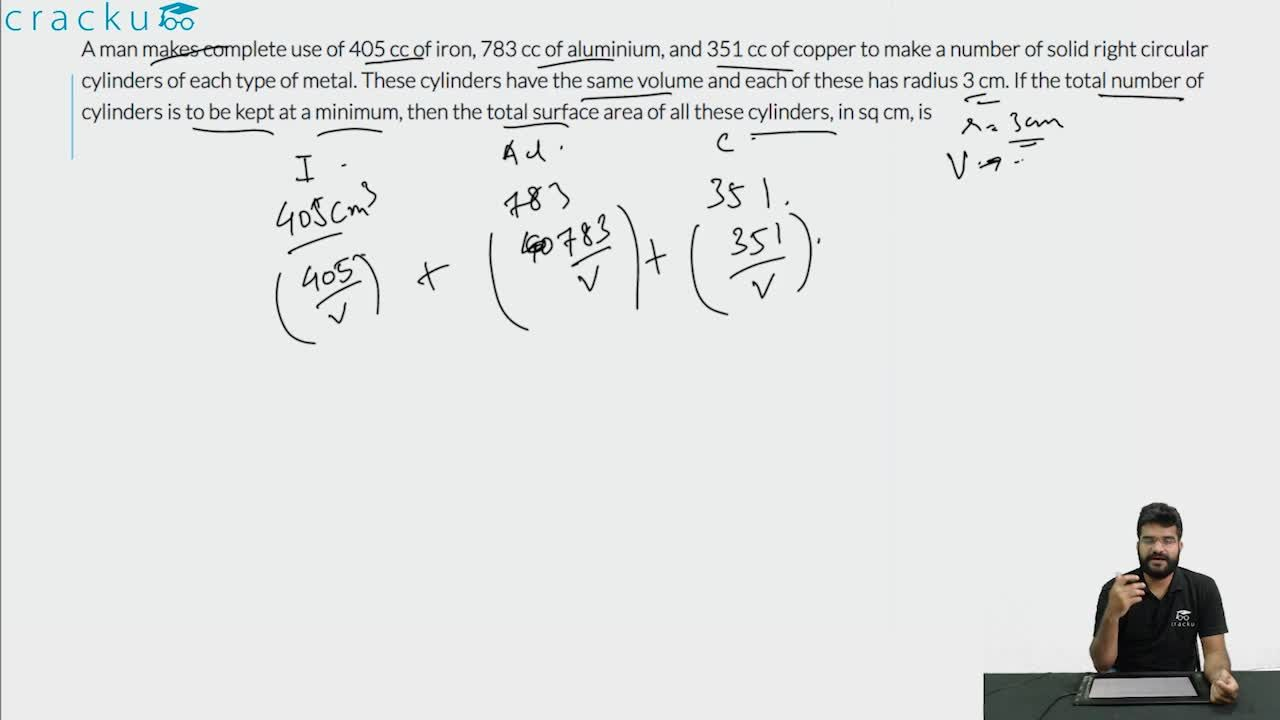Question 82

# A man makes complete use of 405 cc of iron, 783 cc of aluminium, and 351 cc of copper to make a number of solid right circular cylinders of each type of metal. These cylinders have the same volume and each of these has radius 3 cm. If the total number of cylinders is to be kept at a minimum, then the total surface area of all these cylinders, in sq cm, is

Solution

It is given that the volume of all the cylinders is the same, so the volume of each cylinder = HCF of (405, 783, 351)

=27

The number of iron cylinders = $$\ \frac{\ 405}{27}$$ = 15

The number of aluminium cylinders = $$\ \frac{\ 783}{27}$$ = 29

The number of copper cylinders = $$\ \frac{351\ }{27}$$ = 13

15*$$\pi\ r^2h=$$ 405

15*$$\pi\ 9*h=$$ 405

$$\pi\ h=3$$

Now we have to calculate the total surface area of all the cylinders

Total number of cylinders = 15+29+13 = 57

Total surface area of the cylinder = 57*($$2\pi\ rh+2\pi\ r^2$$)

=57(2*3*3 + 2*9*$$\pi\$$)

=$$1026(1 + \pi)$$

### View Video Solution